## Math 122 - Chapter 9 Study Guide

### In Class Portion

1. Evaluate the integral using integration by parts. Look at problems 9.2.1-28*.
2. Evaluate the integral involving a trigonometric function. Look at problems 9.3.1-52*.
3. Evaluate the integral using a trigonometric substitution. Look at problems 9.4.1-26*.
4. Evaluate the integral using partial fractions. Look at problems 9.5.9-32*.
5. Evaluate the improper integral. Look at problems 9.8.3-30*.
6. Evaluate the integral using the table of integrals. The appropriate formula is given to you. Look at problems 9.6.1-24*.

### Notes:

• *'d problems are directly from the text.
• There are NO CALCULATORS allowed on the in-class portion of the exam.
• The take home portion of the exam is worth 70 points and is due the day of the exam.
 # 1 2 3 4 5 6 Total Pts 5 5 5 5 5 5 30

### Take Home Portion (pdf)

Name : ______________________
Exam 3 - Chapter 9, 70 points
Math 122 - Calculus & Analytic Geometry II

1. Find the average water level on the Sangamon River at Illinois Rt 48 in Decatur, IL at for Sept/Oct 2000. Use the URL below and request the "stage" data for 30 days in a tab delimited file. Record the times at midnight on 9/9/2000 through midnight on 10/9/2000 so that you have a full 30 days of information (but 31 endpoints). Also record the water stage at noon for each day from 9/9/2000 to 10/8/2000. You may want to get some of the earlier information recorded now because there are can only show 30 days at a time (unless you fudge with the URL). Record the values in the attached table. (10 points)
http://www-il.usgs.gov/nwis-w/IL/data.components/rt.cgi?statnum=05573540
1. Using the 31 values recorded at midnight, approximate the average water stage using the left-hand endpoints, right-hand endpoints, trapezoidal method, and Simpson's method
2. Using the 30 values recorded at noon, approximate the average water stage using the midpoints
2. Find the following Laplace Transforms (look at problems 9.8.56-57). Note that the Laplace Transform is a function of s only, there is no x in the transform. The restrictions on s that are given in the book are necessary so that the improper integral converges, be sure to state proper restrictions. (10 points)
1.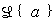2.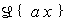3.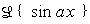4.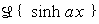5.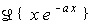3. Work supplemental problem 36 involving the Gamma function. (10 points)

4. Use a table of integrals to find the integral. In each case, copy the integral number and formula and the values of any variables (ex: a or u) (10 points)
1. Problem 9.6.10
2. Problem 9.6.20
3. Problem 9.6.24
4. Problem 9.6.30
5. Problem 9.6.38
5. Use a computer algebra system (Derive, Maple, Mathematica, TI-92) to find the following integrals. (10 points)
1. Problem 9.6.42
2. Problem 9.6.50
3. Problem 9.6.56
4. Problem 9.6.62
5. Problem 9.6.70
6. Work five (5) of the following problems by hand. There must be exactly one problem from each section (9.2, 9.3, 9.4, 9.5, and 9.8). Show all work. Clearly identify the section number and problem. Do not place more than one problem on a sheet of paper and attach the problems in section order. You may use the reduction formulas where necessary, but otherwise do not use the table of integrals. You may use a CAS to check your answer, but show work. (20 points)
1. Problem 9.2.8
2. Problem 9.2.38
3. Problem 9.3.8
4. Problem 9.3.34
5. Problem 9.4.12
6. Problem 9.4.18
7. Problem 9.4.40
8. Problem 9.5.20
9. Problem 9.5.24
10. Problem 9.8.8
11. Problem 9.8.27
12. Problem 9.8.42

70% of the test is take home. You may work the first three problems in groups of up to three and turn in one paper per group, but each student's exam should contain the answers to the rest of the problems. Be sure you double check and understand the work of those in your group, it's your grade, too.